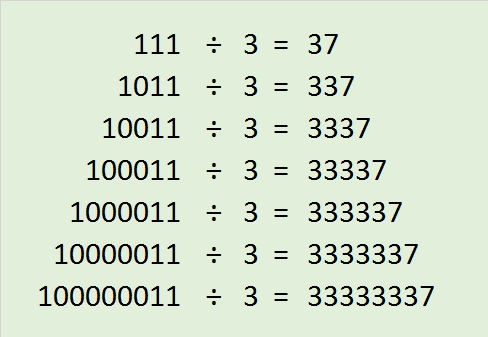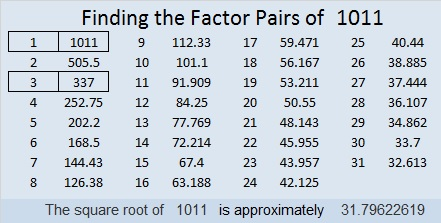# 1011 A Toast to My Readers

Thank you, dear readers, for stopping by today and/or any other time you have visited! My blog had the best year ever in 2017, and it is all because of you. I sincerely hope that I have been of service to you.

Here’s to you, dear friend. May lifting each other help us both to climb even higher this coming year!

May you be able to find the factors in this puzzle and, more importantly, the factors in life that will bring you happiness and success!Print the puzzles or type the solution in this excel file: 10-factors-1002-1011

Let me tell you some things about the number 1011 that you probably didn’t know before:

The only nonzero digits in 1011 are three 1’s so 1011 is divisible by 3, and 1011 is included in this interesting pattern:1011² = 1022121; Notice that the digits in bold are 1011 and all the other digits are 2’s. Thank you OEIS.org for that fun fact.

1011 looks interesting in a few other bases:
It’s 33303 in BASE 4 because 3(4⁴ + 4³ + 4² + 4⁰) = 3(256 + 64 + 16 + 1) = 3(337) = 1011,
323 in BASE 18 because 3(18²) + 2(18) + 3(1) = 1011

1011 is the hypotenuse of a Pythagorean triple:
525-864-1011 which is 3 times (175-288-337)

• 1011 is a composite number.
• Prime factorization: 1011 = 3 × 337
• The exponents in the prime factorization are 1 and 1. Adding one to each and multiplying we get (1 + 1)(1 + 1) = 2 × 2 = 4. Therefore 1011 has exactly 4 factors.
• Factors of 1011: 1, 3, 337, 1011
• Factor pairs: 1011 = 1 × 1011 or 3 × 337
• 1011 has no square factors that allow its square root to be simplified. √1011 ≈ 31.7962